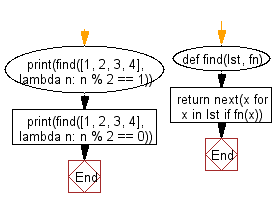﻿ Python: Find the value of the first element in the given list that satisfies the provided testing function - w3resource# Python: Find the value of the first element in the given list that satisfies the provided testing function

## Python List: Exercise - 239 with Solution

Write a Python program to find the value of the first element in the given list that satisfies the provided testing function.

Use a list comprehension and next() to return the first element in lst for which fn returns True.

Sample Solution:

Python Code:

``````def find(lst, fn):
return next(x for x in lst if fn(x))
print(find([1, 2, 3, 4], lambda n: n % 2 == 1))
print(find([1, 2, 3, 4], lambda n: n % 2 == 0))
```
```

Sample Output:

```1
2
```

Flowchart:## Visualize Python code execution:

The following tool visualize what the computer is doing step-by-step as it executes the said program:

Python Code Editor:

Have another way to solve this solution? Contribute your code (and comments) through Disqus.

What is the difficulty level of this exercise?

Test your Python skills with w3resource's quiz

﻿

## Python: Tips of the Day

Floor Division:

When we speak of division we normally mean (/) float division operator, this will give a precise result in float format with decimals.

For a rounded integer result there is (//) floor division operator in Python. Floor division will only give integer results that are round numbers.

```print(1000 // 300)
print(1000 / 300)```

Output:

```3
3.3333333333333335```